# RS Aggarwal Solutions for Class 12 Maths Chapter 13: Methods of Integration Exercise 13B

In this exercise, the problems are based on the concept of integration using trigonometric identities. Students can solve the exercise wise problems more easily using the solutions PDF as a reference guide. Using the solutions while solving problems improves the analytical thinking and problem solving abilities among students. Students can boost their confidence in solving the exercise wise problems by using RS Aggarwal Solutions Class 12 Maths Chapter 13 Methods of Integration Exercise 13B PDF from the links, which are available here.

## RS Aggarwal Solutions for Class 12 Maths Chapter 13: Methods of Integration Exercise 13B Download PDF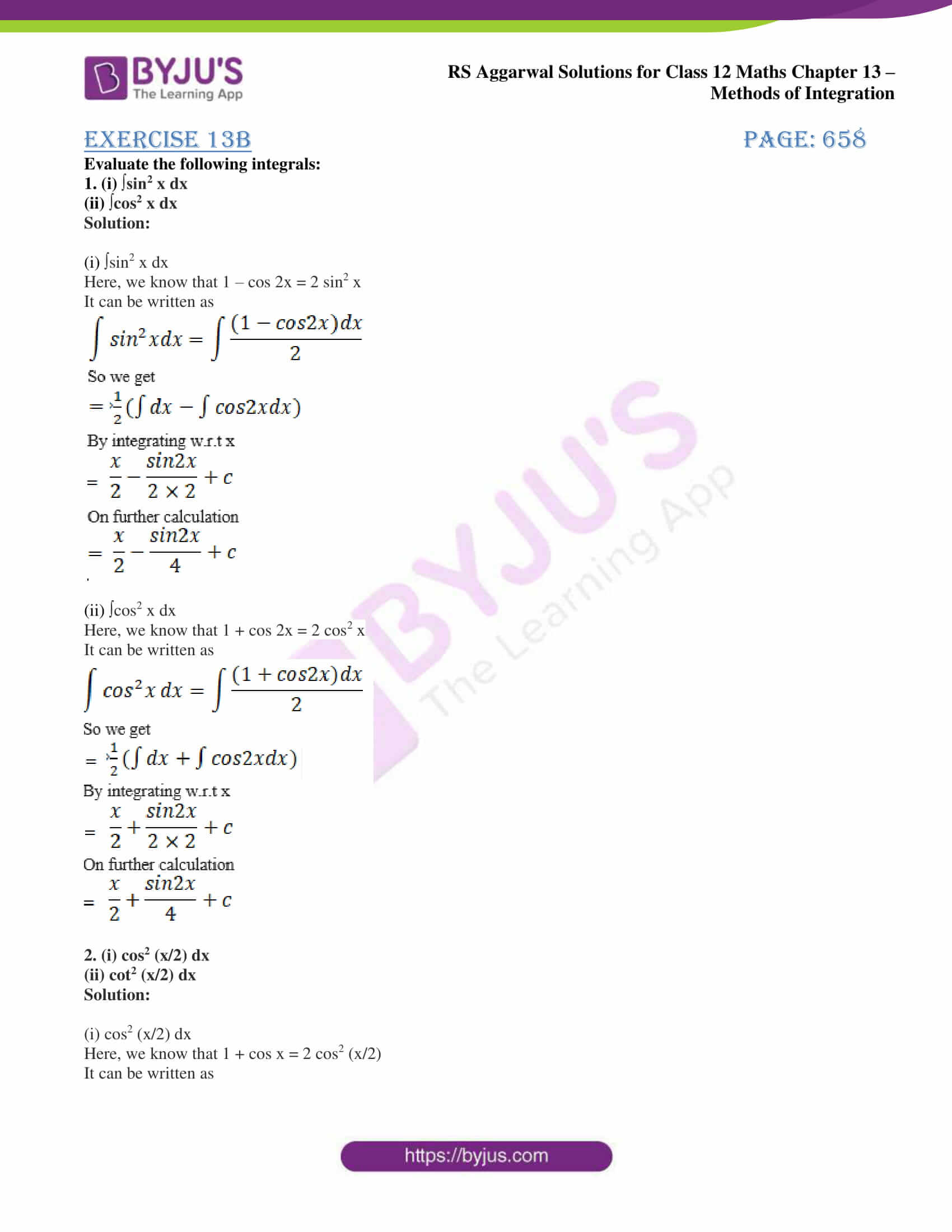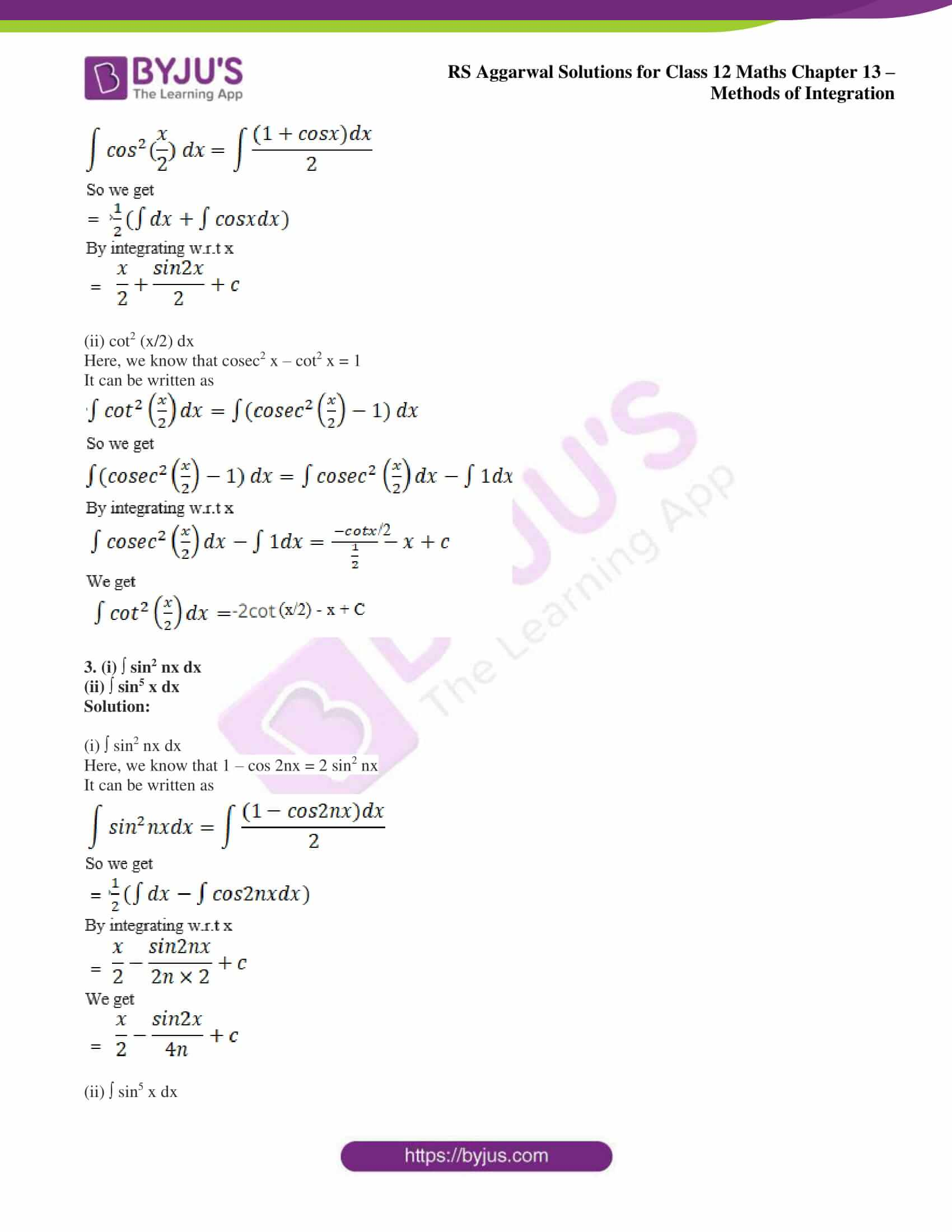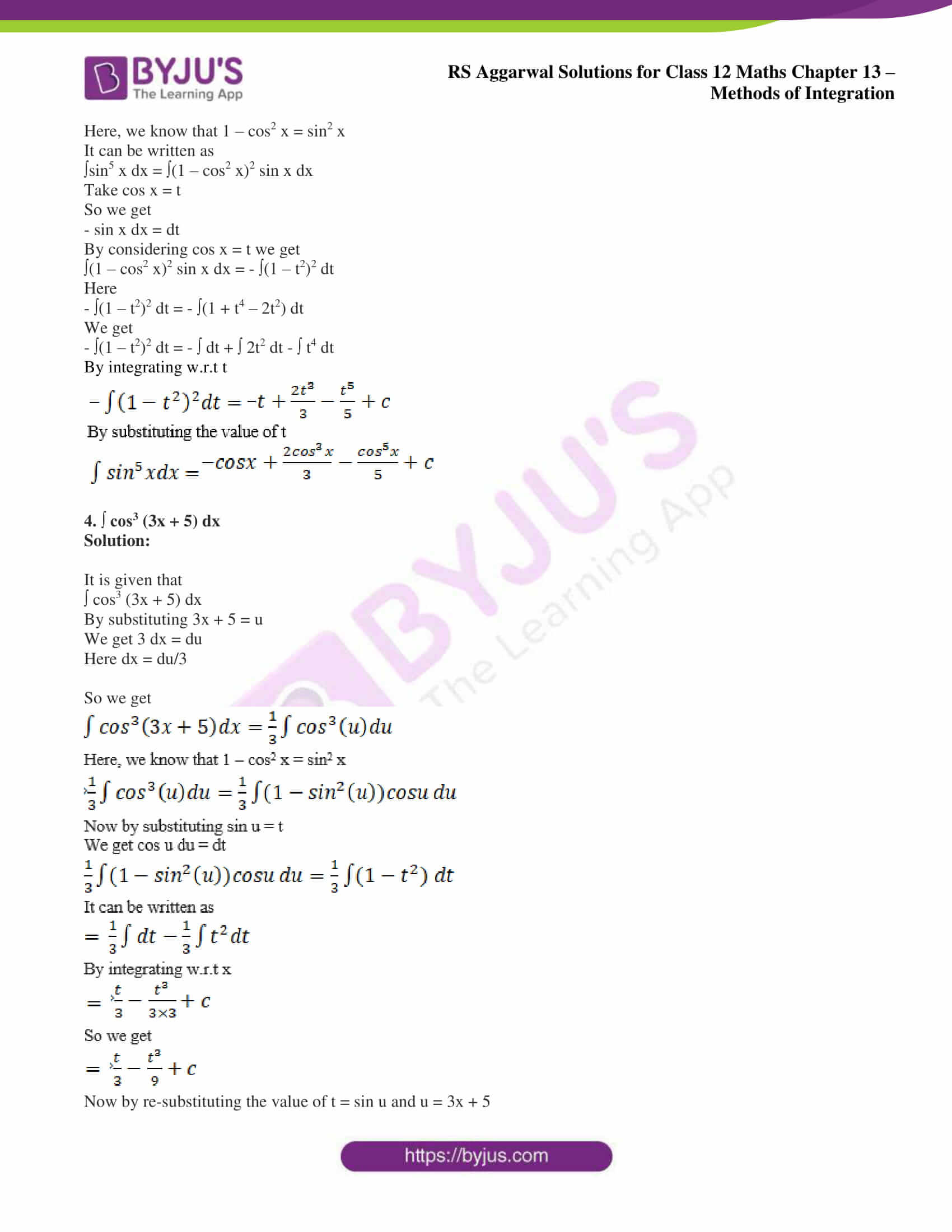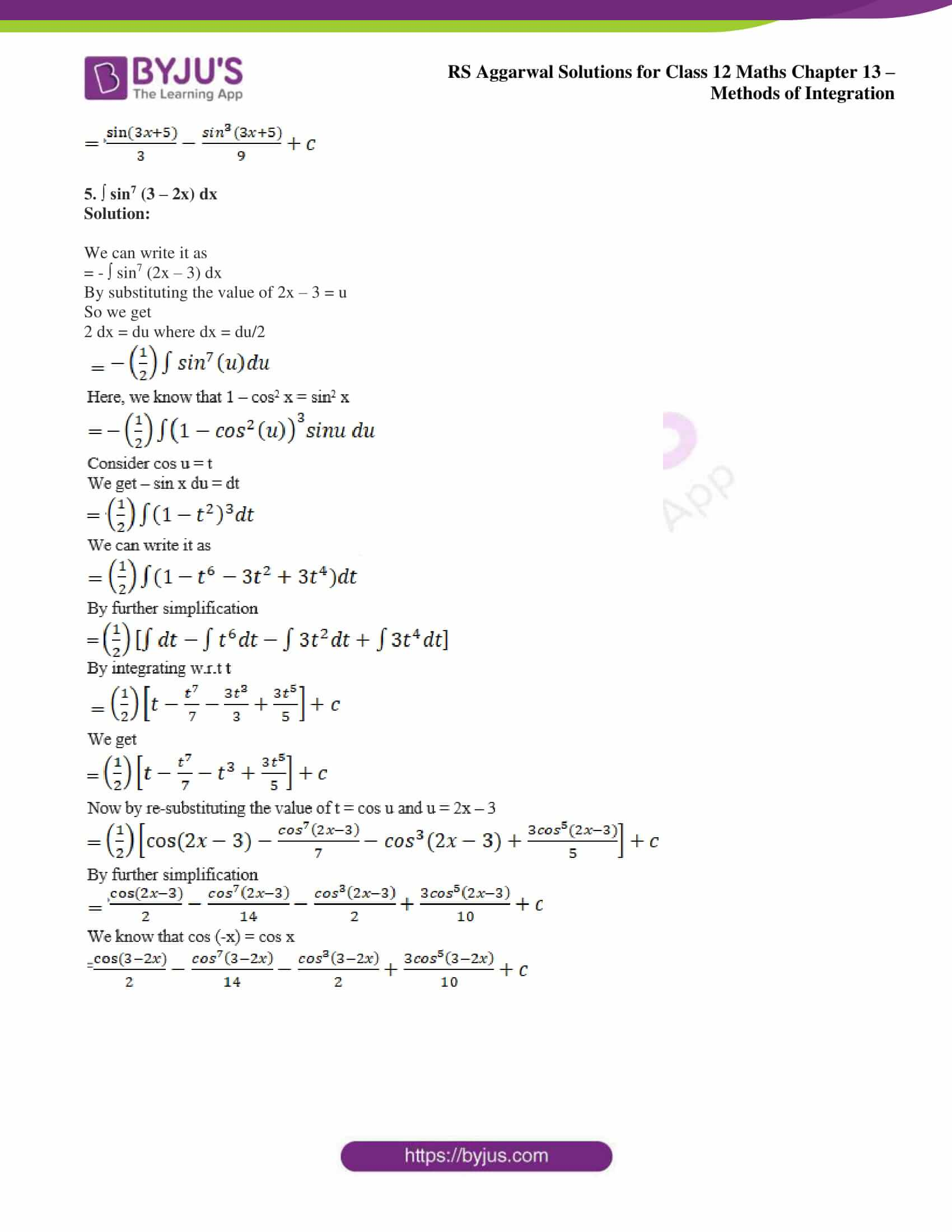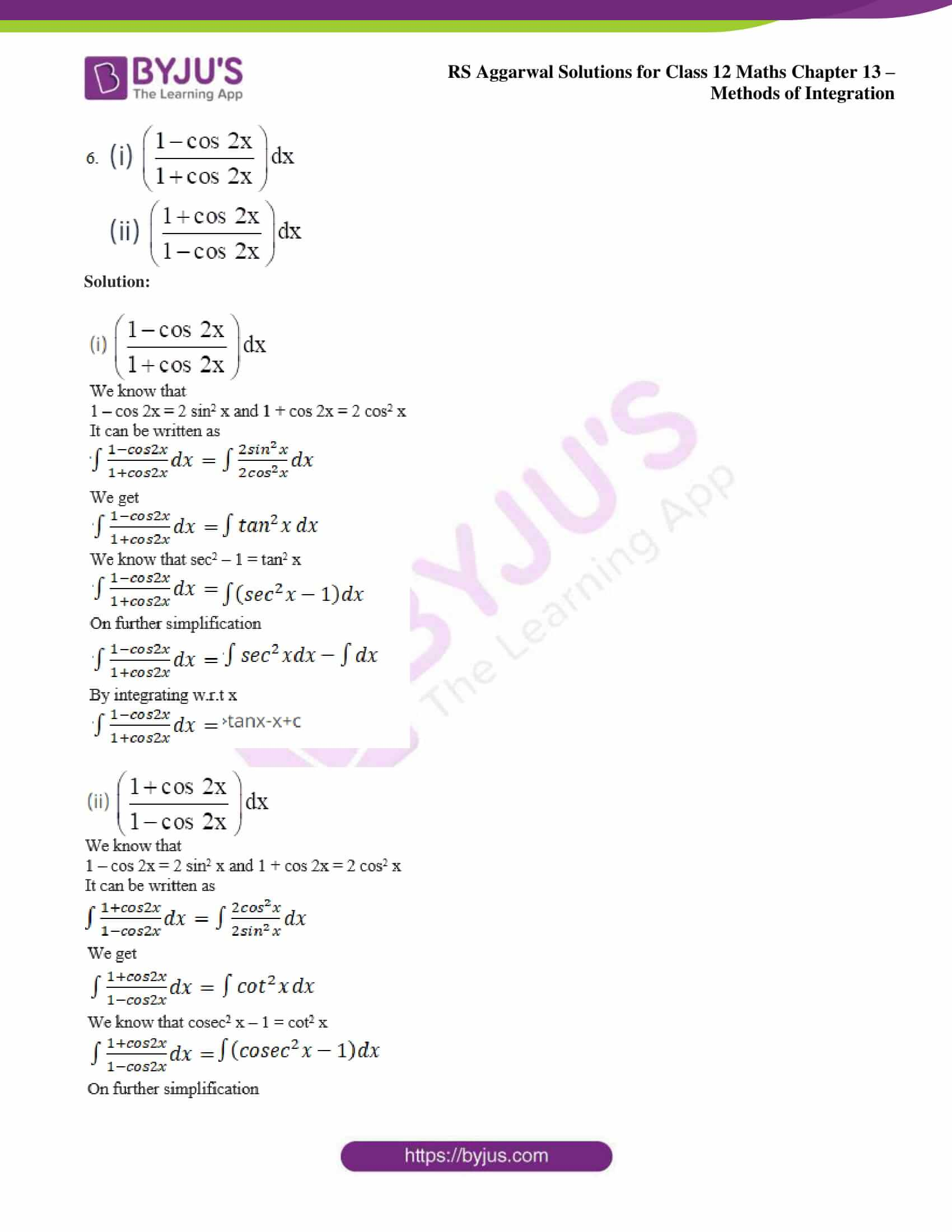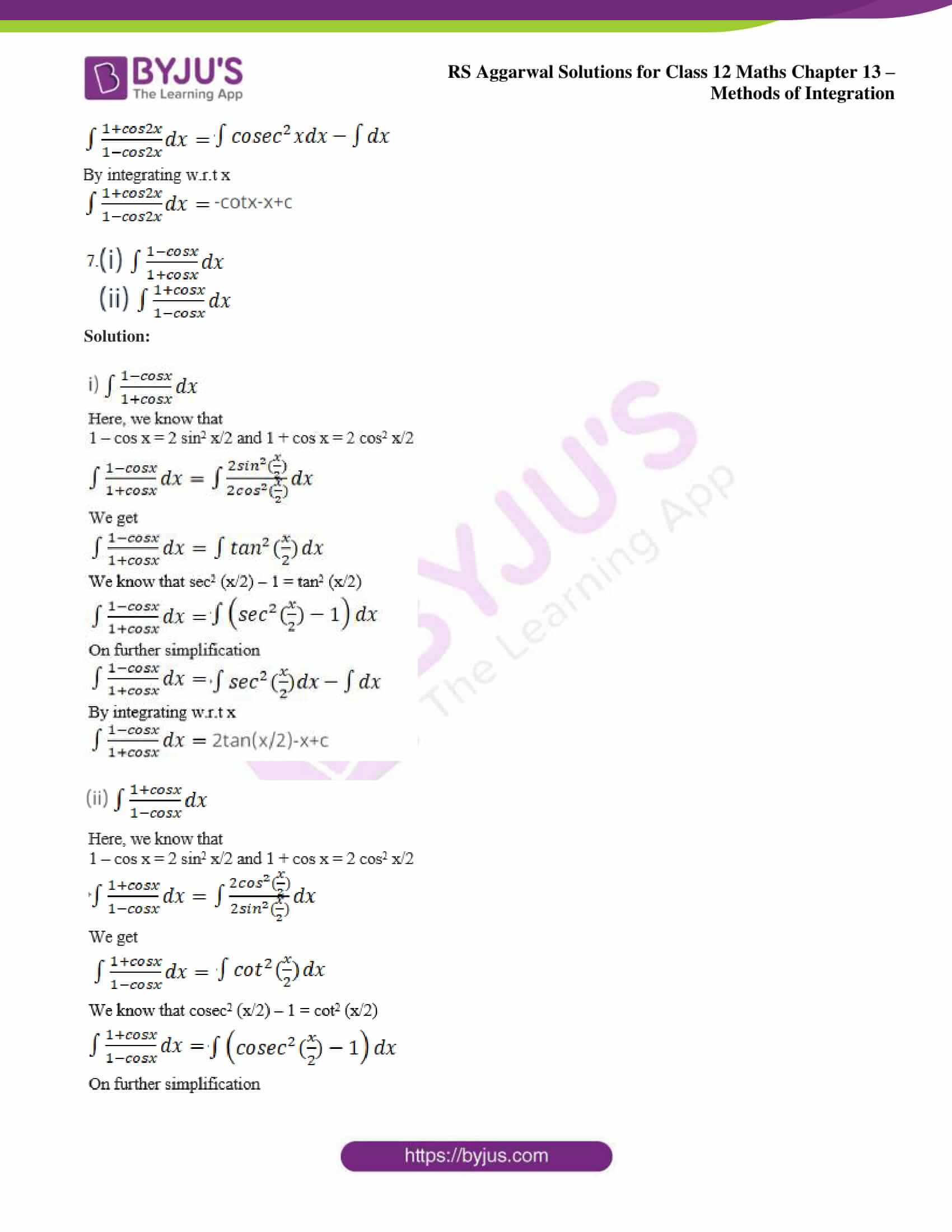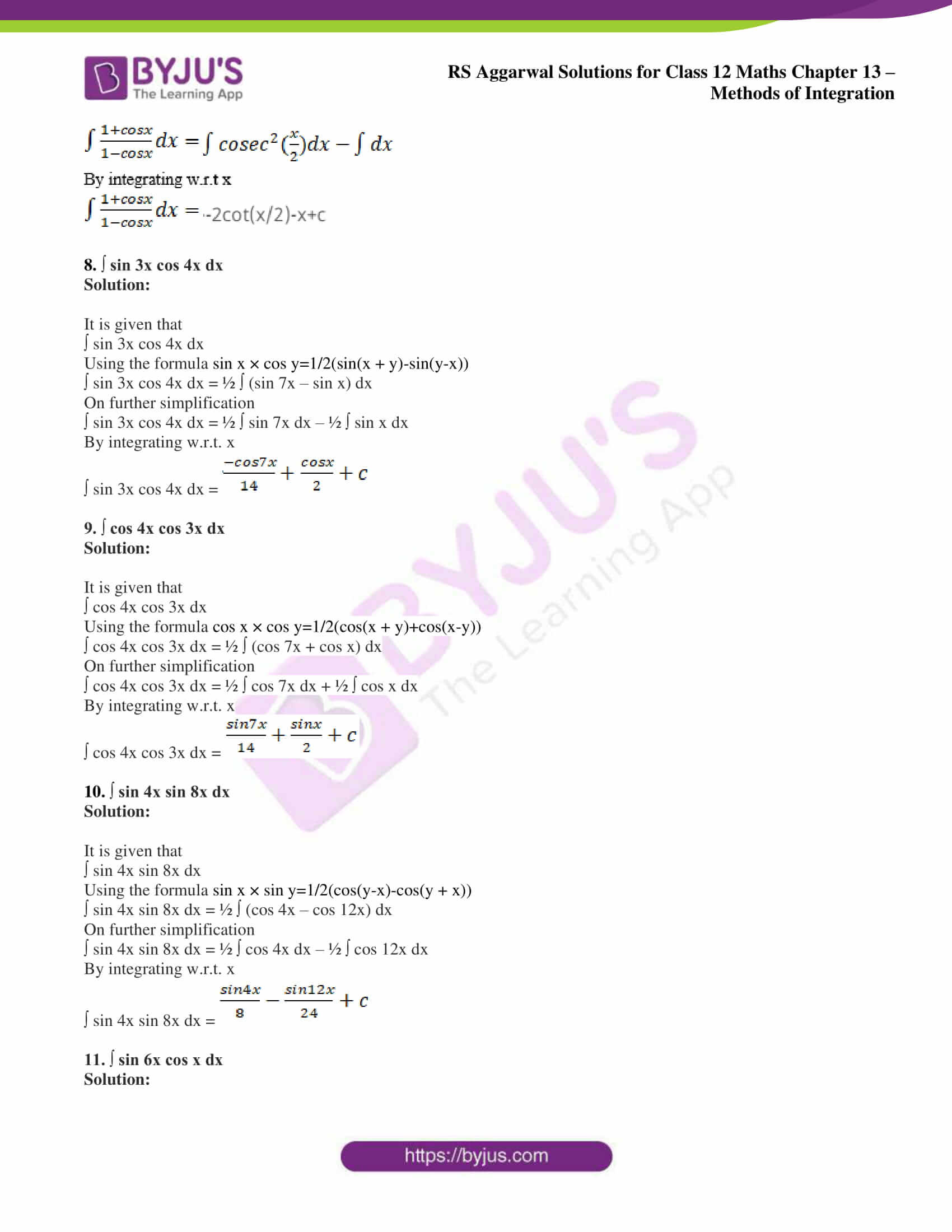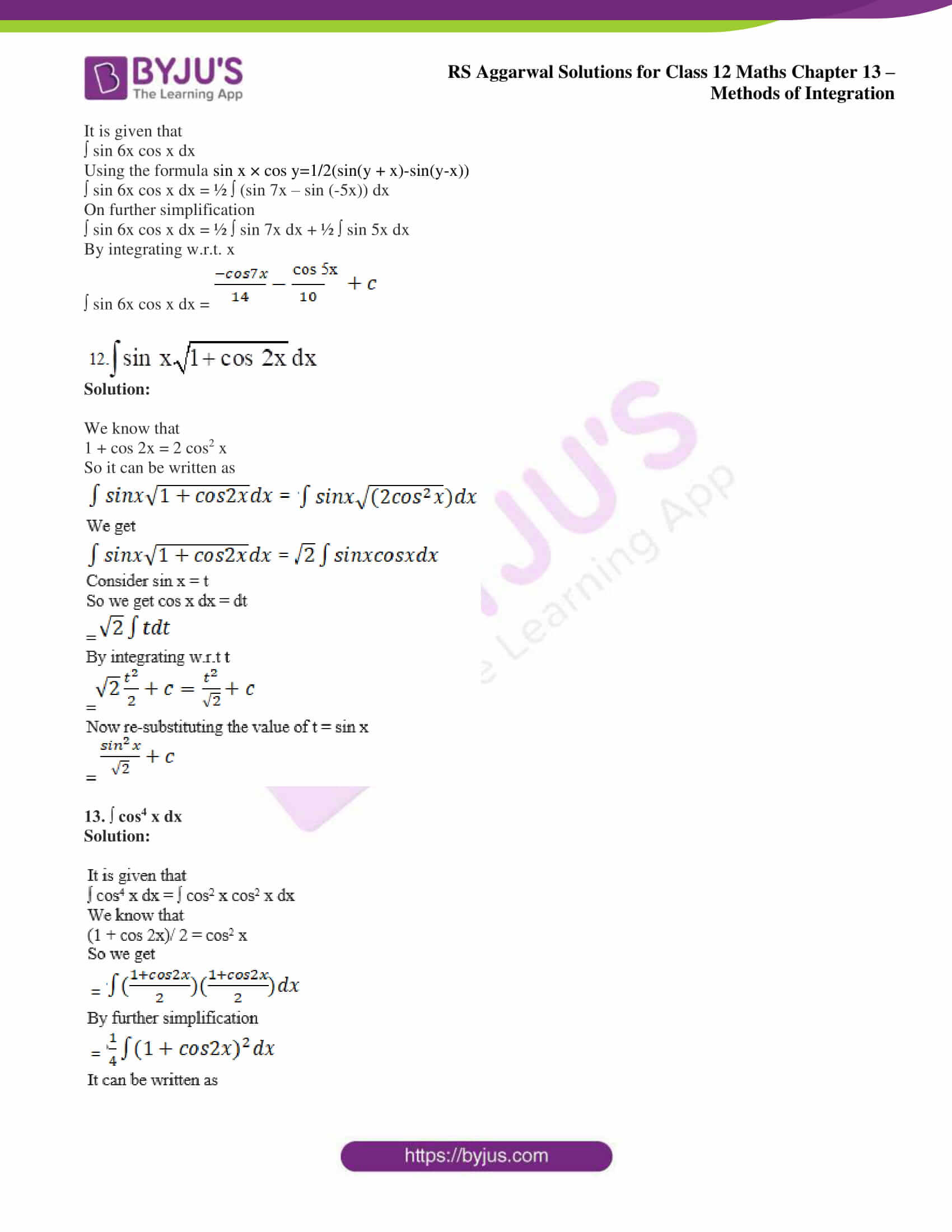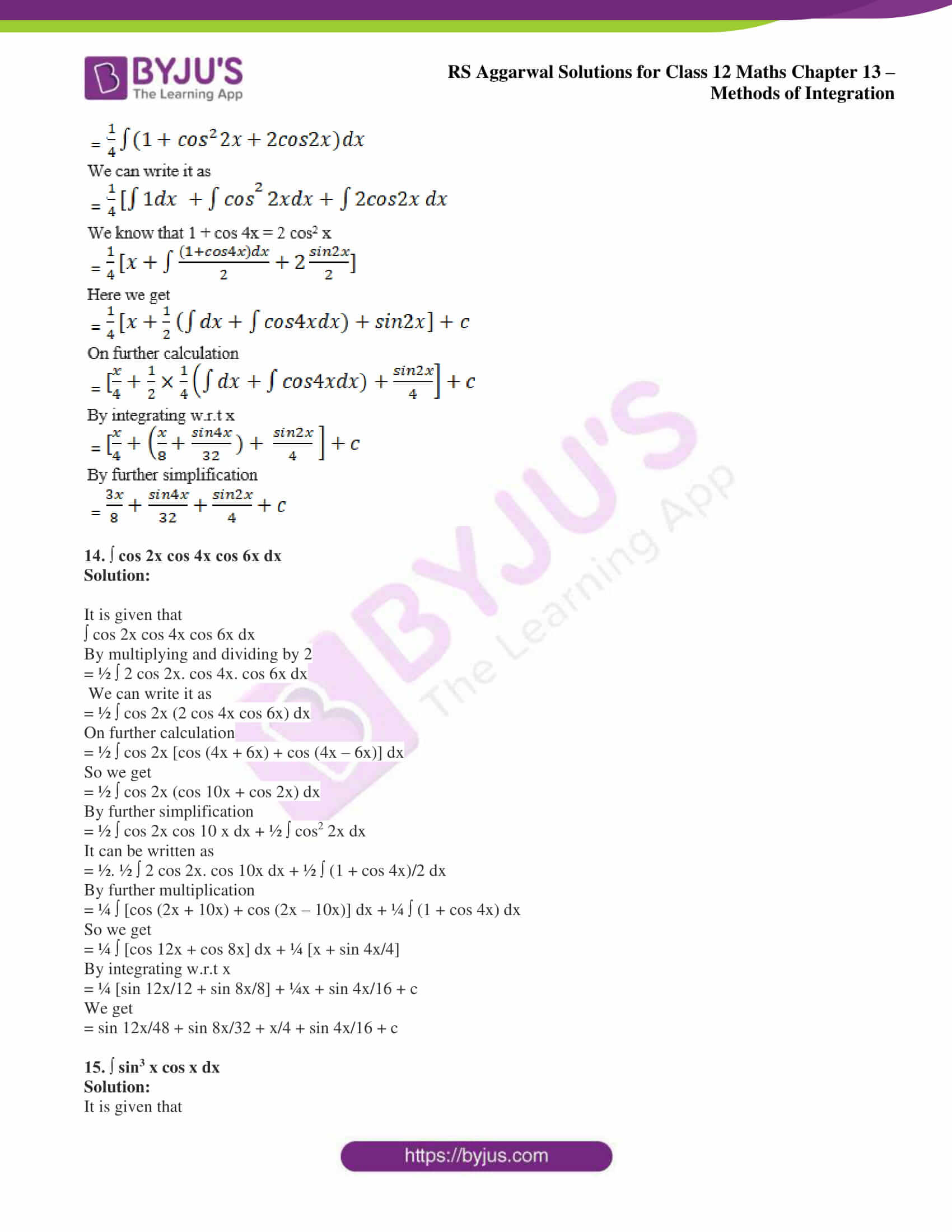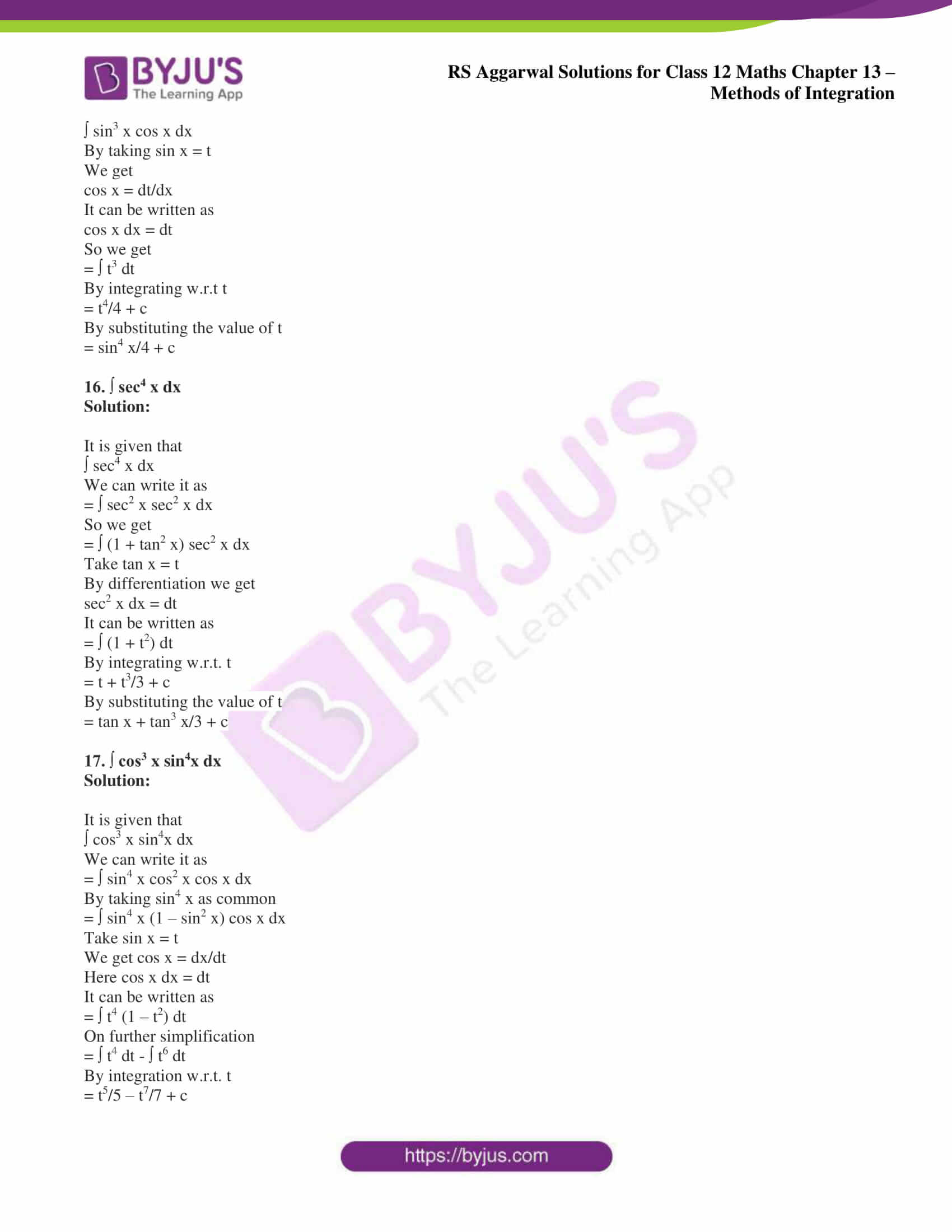### Access other exercise solutions of Class 12 Maths Chapter 13: Methods of Integration

Exercise 13A Solutions

Exercise 13C Solutions

### Access RS Aggarwal Solutions for Class 12 Maths Chapter 13: Methods of Integration Exercise 13B

Evaluate the following integrals:

1. (i) ∫sin2 x dx

(ii) ∫cos2 x dx

Solution:

(i) ∫sin2 x dx

Here, we know that 1 – cos 2x = 2 sin2 x

It can be written as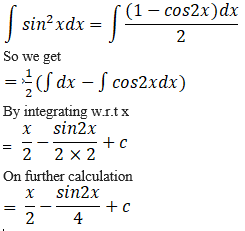(ii) ∫cos2 x dx

Here, we know that 1 + cos 2x = 2 cos2 x

It can be written as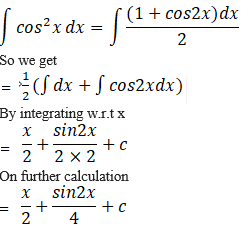2. (i) cos2 (x/2) dx

(ii) cot2 (x/2) dx

Solution:

(i) cos2 (x/2) dx

Here, we know that 1 + cos x = 2 cos2 (x/2)

It can be written as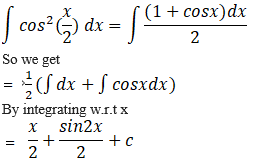(ii) cot2 (x/2) dx

Here, we know that cosec2 x – cot2 x = 1

It can be written as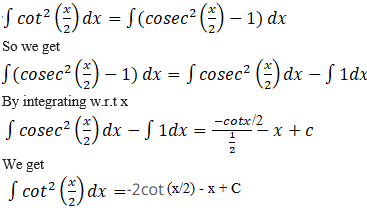3. (i) ∫ sin2 nx dx

(ii) ∫ sin5 x dx

Solution:

(i) ∫ sin2 nx dx

Here, we know that 1 – cos 2nx = 2 sin2 nx

It can be written as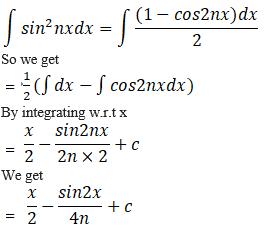(ii) ∫ sin5 x dx

Here, we know that 1 – cos2 x = sin2 x

It can be written as

∫sin5 x dx = ∫(1 – cos2 x)2 sin x dx

Take cos x = t

So we get

– sin x dx = dt

By considering cos x = t we get

∫(1 – cos2 x)2 sin x dx = – ∫(1 – t2)2 dt

Here

– ∫(1 – t2)2 dt = – ∫(1 + t4 – 2t2) dt

We get

– ∫(1 – t2)2 dt = – ∫ dt + ∫ 2t2 dt – ∫ t4 dt

By integrating w.r.t t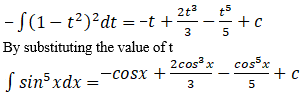4. ∫ cos3 (3x + 5) dx

Solution:

It is given that

∫ cos3 (3x + 5) dx

By substituting 3x + 5 = u

We get 3 dx = du

Here dx = du/3

So we get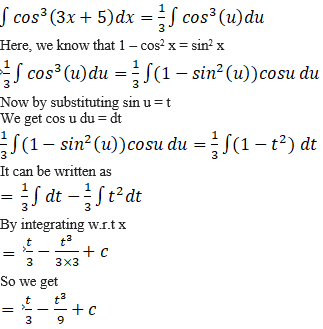Now by re-substituting the value of t = sin u and u = 3x + 5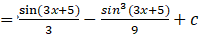5. ∫ sin7 (3 – 2x) dx

Solution:

We can write it as

= – ∫ sin7 (2x – 3) dx

By substituting the value of 2x – 3 = u

So we get

2 dx = du where dx = du/2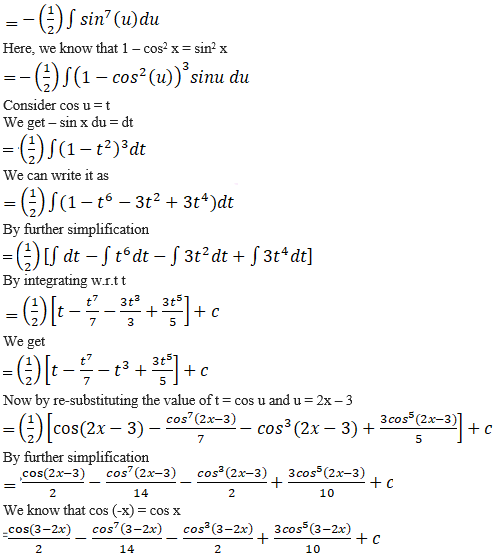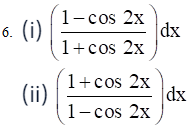Solution: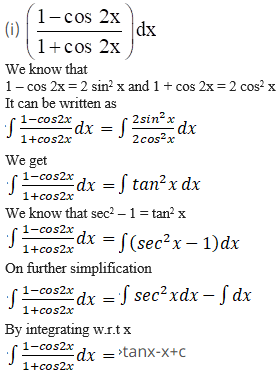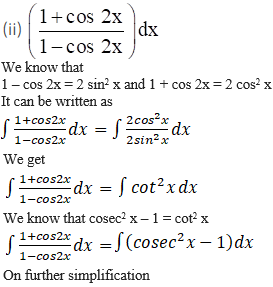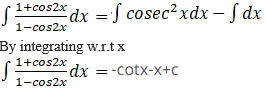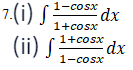Solution: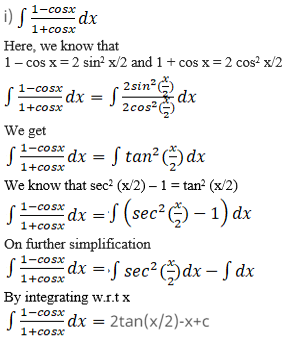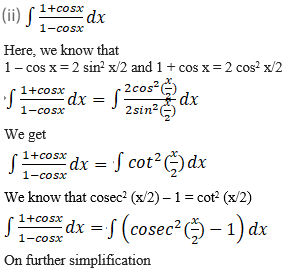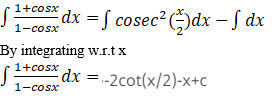8. ∫ sin 3x cos 4x dx

Solution:

It is given that

∫ sin 3x cos 4x dx

Using the formula sin x × cos y=1/2(sin(x + y)-sin(y-x))

∫ sin 3x cos 4x dx = ½ ∫ (sin 7x – sin x) dx

On further simplification

∫ sin 3x cos 4x dx = ½ ∫ sin 7x dx – ½ ∫ sin x dx

By integrating w.r.t. x

∫ sin 3x cos 4x dx =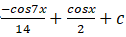9. ∫ cos 4x cos 3x dx

Solution:

It is given that

∫ cos 4x cos 3x dx

Using the formula cos x × cos y=1/2(cos(x + y)+cos(x-y))

∫ cos 4x cos 3x dx = ½ ∫ (cos 7x + cos x) dx

On further simplification

∫ cos 4x cos 3x dx = ½ ∫ cos 7x dx + ½ ∫ cos x dx

By integrating w.r.t. x

∫ cos 4x cos 3x dx =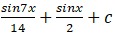10. ∫ sin 4x sin 8x dx

Solution:

It is given that

∫ sin 4x sin 8x dx

Using the formula sin x × sin y=1/2(cos(y-x)-cos(y + x))

∫ sin 4x sin 8x dx = ½ ∫ (cos 4x – cos 12x) dx

On further simplification

∫ sin 4x sin 8x dx = ½ ∫ cos 4x dx – ½ ∫ cos 12x dx

By integrating w.r.t. x

∫ sin 4x sin 8x dx =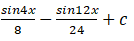11. ∫ sin 6x cos x dx

Solution:

It is given that

∫ sin 6x cos x dx

Using the formula sin x × cos y=1/2(sin(y + x)-sin(y-x))

∫ sin 6x cos x dx = ½ ∫ (sin 7x – sin (-5x)) dx

On further simplification

∫ sin 6x cos x dx = ½ ∫ sin 7x dx + ½ ∫ sin 5x dx

By integrating w.r.t. x

∫ sin 6x cos x dx =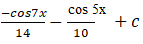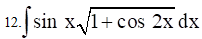Solution:

We know that

1 + cos 2x = 2 cos2 x

So it can be written as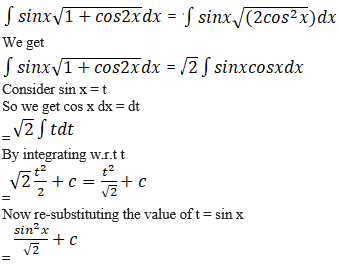13. ∫ cos4 x dx

Solution: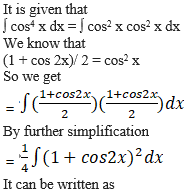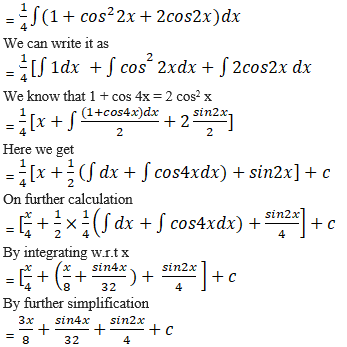14. ∫ cos 2x cos 4x cos 6x dx

Solution:

It is given that

∫ cos 2x cos 4x cos 6x dx

By multiplying and dividing by 2

= ½ ∫ 2 cos 2x. cos 4x. cos 6x dx

We can write it as

= ½ ∫ cos 2x (2 cos 4x cos 6x) dx

On further calculation

= ½ ∫ cos 2x [cos (4x + 6x) + cos (4x – 6x)] dx

So we get

= ½ ∫ cos 2x (cos 10x + cos 2x) dx

By further simplification

= ½ ∫ cos 2x cos 10 x dx + ½ ∫ cos2 2x dx

It can be written as

= ½. ½ ∫ 2 cos 2x. cos 10x dx + ½ ∫ (1 + cos 4x)/2 dx

By further multiplication

= ¼ ∫ [cos (2x + 10x) + cos (2x – 10x)] dx + ¼ ∫ (1 + cos 4x) dx

So we get

= ¼ ∫ [cos 12x + cos 8x] dx + ¼ [x + sin 4x/4]

By integrating w.r.t x

= ¼ [sin 12x/12 + sin 8x/8] + ¼x + sin 4x/16 + c

We get

= sin 12x/48 + sin 8x/32 + x/4 + sin 4x/16 + c

15. ∫ sin3 x cos x dx

Solution:

It is given that

∫ sin3 x cos x dx

By taking sin x = t

We get

cos x = dt/dx

It can be written as

cos x dx = dt

So we get

= ∫ t3 dt

By integrating w.r.t t

= t4/4 + c

By substituting the value of t

= sin4 x/4 + c

16. ∫ sec4 x dx

Solution:

It is given that

∫ sec4 x dx

We can write it as

= ∫ sec2 x sec2 x dx

So we get

= ∫ (1 + tan2 x) sec2 x dx

Take tan x = t

By differentiation we get

sec2 x dx = dt

It can be written as

= ∫ (1 + t2) dt

By integrating w.r.t. t

= t + t3/3 + c

By substituting the value of t

= tan x + tan3 x/3 + c

17. ∫ cos3 x sin4x dx

Solution:

It is given that

∫ cos3 x sin4x dx

We can write it as

= ∫ sin4 x cos2 x cos x dx

By taking sin4 x as common

= ∫ sin4 x (1 – sin2 x) cos x dx

Take sin x = t

We get cos x = dx/dt

Here cos x dx = dt

It can be written as

= ∫ t4 (1 – t2) dt

On further simplification

= ∫ t4 dt – ∫ t6 dt

By integration w.r.t. t

= t5/5 – t7/7 + c

By substituting the value of t

= sin5 x/5 – sin7 x/7 + c